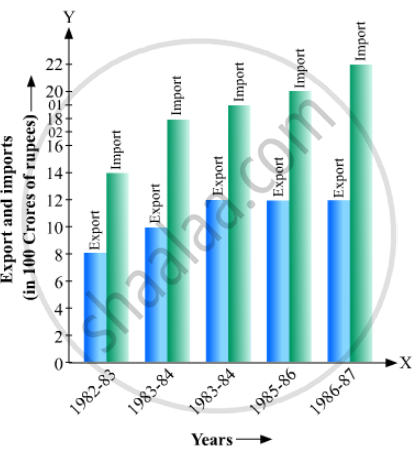# Read the Following Bar Graph and Answer the Following Questions: (I) What Information is Given by the Bar Graph? (Ii) in Which Year the Export is Minimum? (Iii)In Which Year the Import is Maximum? - Mathematics

(i) What information is given by the bar graph?
(ii) In which year the export is minimum?
(iii)In which year the import is maximum?
(iv)In which year the difference of the values of export and import is maximum?#### Solution

(1) The bar graph represents the import and export (in 100 Crores of rupees) from 1982-83 to 1986-87.

(2) The export is minimum in the year 1982-83 as the height of the bar corresponding to export is minimum in the year 1982-83.

(3) The import is maximum in the year 1986-87 as the height of the bar corresponding to import is maximum in the year 1986-87.

(4) The bars of export and import are side by side. Clearly, it is seen from the bar graph that the difference between the values of export and import is maximum in the year 1986-87.

Concept: Graphical Representation of Data
Is there an error in this question or solution?

#### APPEARS IN

RD Sharma Mathematics for Class 9
Chapter 23 Graphical Representation of Statistical Data
Exercise 23.2 | Q 2 | Page 23

Share